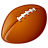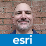# Is Densified Geometry a Circle

343
4
04-02-2018 02:29 PMby
Occasional Contributor III

I create circles in my project using the out of the box circle tool, but then I densify those circles immediately after they are created.  But know I need to know if that densified shape that i created is a circle?  Is there a way to get all the points of that densified polygon and see if they are all equidistant apart.  This would tell me that the polygon is a densified circle.

4 RepliesbyMVP Legendary Contributor

A circle in any implementation by esri that I have seen is an 'n-gon' a 36 (or other multiple) sided shape on the perimeter of a circle.  The points on that circle are connected by straight lines, so densifying them simply adds point(s) on the straight line and not at points of the same distance from the centre that the original points were.  In that context, densifying the circle does little (nothing) to improve the appearance of circularity.

If you are concerned about the points being equidistant from the center radiating at some specified angle (ie 0.5 or 1 degree) increment, then you can generate them yourself.  Implementations exist on geonet (ie python)by
Occasional Contributor III

So, then I just need to know if a shape is a circle.  If what you say is correct, that densify just adds more vertices, then is there some method to determine if the shape is a representation of a circle?byMVP Legendary Contributor

Calculate the centroid of the polygon, convert its boundary to points and determine the center to point distances... circles will have radii all the same (within calculation limits)

Or determine the perimeter/area ratio. the standard test for circularity since perimeter, area and radius (diameter) are all inter-related.byEsri Esteemed Contributor

A circle will have a ratio for area to perimeter that is based on the radius ((pi * radius * radius) / (2 * pi * radius) == radius/2).

Unfortunately, there are plenty of caveats:

• If area is *exactly* "pi-r-squared", the object was likely originally captured by ArcGIS as a circle, and has CAD geometry to remember, even if the vertex count is low.
• If the object wasn't a CAD circle, then the closest you will achieve is "circle-ish"
• Reprojected circles are unlikely to be circular in decimal degrees, so you may need to project geographic coordinate system coordinate values before evaluating them

- V